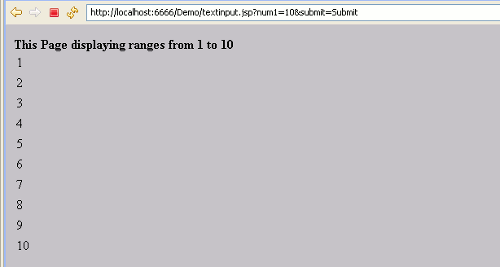Tutorials

# JSP for loop Example

The for loop is a control flow statement, which allows code to be executed repeatedly based on the given condition.

The for loop is a control flow statement, which allows code to be executed repeatedly based on the given condition.

## JSP for loop Example

In this section we will discuss about for loop in JSP. In every programming languages for loop is used. The for loop statement provide a way to iterate over a range of values. Now here is the example which will illustrate how to use for loop in JSP.

Example : In this example we are going to create JSP page named "firstpage.jsp" in which we have created one text box. When the user enter any number in the textbox, it will go to another JSP page named  "textinput.jsp". Using for loop we will iterate it up to the number entered in the textbox. And using request.getParameter(), it will  read the value from textbox . So, using Integer.parseInt() convert the value of textbox  into integer. After that print the value of i, it will prints from 1 to the number entered in the text box. There is also a validation in the form, that if you have not entered any value in the textbox then it will display the message "Sorry you have not entered any value". Here is the firstpage.jsp code.

```<%@ page contentType="text/html; charset=iso-8859-1" language="java" %>
<html><script type="text/javascript">
function check()
{
var x=document.forms["frm"]["num1"].value;
if (x==null || x=="")
{
alert("Sorry You have not entered any value");
return false;
}
return true;

}
</script><title>JSP For-Loop Example</title>
<body bgcolor="#6E4E6E">
<form name="frm" method="get" action="textinput.jsp" onsubmit="check()">
<table width="100%" border="0" cellspacing="0" cellpadding="0" bgcolor="#D8D8D8 ">
<p><font color="#F8E0F7"></font></p>
<tr>
<td width="22%">&nbsp;</td>
<td width="78%">&nbsp;</td>
</tr>
<tr><center>
<td>Enter the number:</td>
<td><input type="text" name="num1"></td>
</tr>
<tr>
<td>&nbsp;</td>
<td><input type="submit" name="submit" value="Submit"></td>
</tr>
<tr></center>
<td>&nbsp;</td>
<td>&nbsp;</td>
</tr>
</table>
</form>
</body>
</html>```

And, here is textinput.jsp page

```<!DOCTYPE html PUBLIC "-//W3C//DTD HTML 4.01 Transitional//EN" "http://www.w3.org/TR/html4/loose.dtd">
<%@ page contentType="text/html; charset=iso-8859-1" language="java" %>

<html>
<body bgcolor="#C0C0C0">
<%String a=request.getParameter("num1");%>

<br/><%int number=Integer.parseInt(a);
for(int i=1;i<=number;i++)
{
%>
<table border="0"><tr><td><%=i %></td></tr></table>
<%} %>
</body>
</html>
```

You can see the code above for the program which we have created. When you execute the program the output will be as follows:When you enter some value, then it will display the number like below: